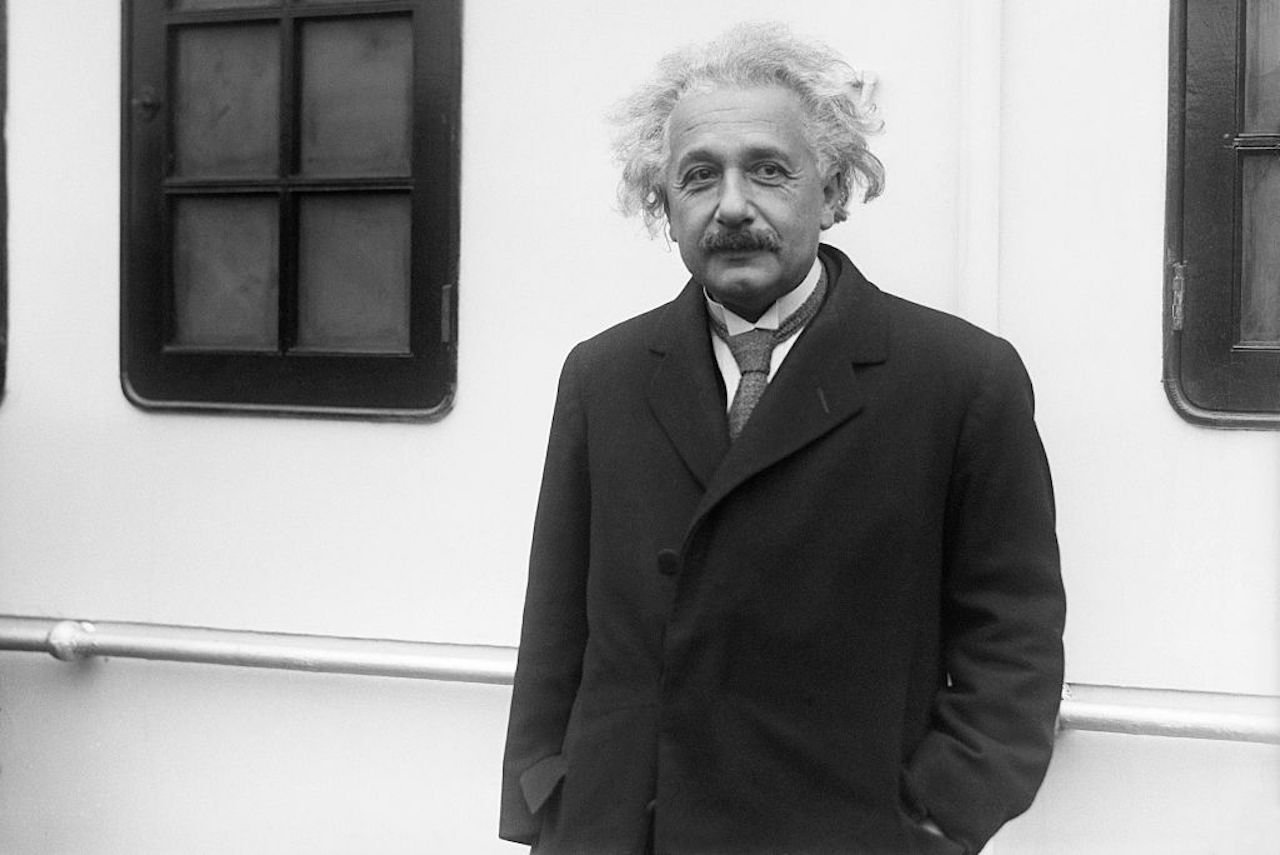HomeFrom our readersAlbert Einstein’s Pi Day Birthday is Special

# Albert Einstein’s Pi Day Birthday is Special# Albert Einstein’s Pi Day Birthday is Special

From Aziz Inan, professor, University of Portland

Albert Einstein who was born on Mar.14 1879 in Ulm, Germany and died on Apr.18, 1955 in Princeton, NJ, was a German-born theoretical physicist, widely acknowledged to be one of the greatest physicists of all time.

Einstein is best known for his theory of relativity. Additionally, he made significant contributions to quantum mechanics. Einstein is also known for his mass–energy equivalence formula E=mc^2. In 1921, the Nobel Prize in Physics was awarded to Albert Einstein “for his services to Theoretical Physics, and especially for his discovery of the law of the photoelectric effect,” which played a pivotal step in the development of quantum theory.

March 14, 2022 marks Einstein’s 143rd birthday and I prepared the following numerical birthday curiosities in his honor:

1. Einstein’s birthday number 143 interpreted in day/month date format as 14/3 coincides with his birthdate.

2. Also, 143 equals 76 plus its reverse, namely 67. Interestingly, Einstein died 67 years ago, at age 76.

3. Further, the sum of the prime factors of 143, namely 11 and 13, equals 24 and the reverse of 24, namely 42, equals 14×3.

4. Einstein’s birthdate coincides with Pi Day (03/14). Interestingly, the reverse of 0314, namely 4130, equals 2x5x7x59 and the sum of these prime factors equals 73. Interestingly, the 73rd day of any non-leap year is Pi Day.

5. The sum of 14 and 3, the day and month numbers of Einstein’s birthdate, equals 17 and 17 square is 289. Interestingly, the 289th prime number is 1879, Einstein’s birth year.

6. Einstein’s birthday 3/14 is the 73rd day of each non-leap year. Interestingly, 73 times the difference of its digits, namely 4, equals 292 and 292 is the number of days left in each year after his birthday.

7. Einstein died in 1955 on 18/04 and the prime factors of 1804 add up to 2+11+41=54. Interestingly, twice 54 equals 108 and the 108th day of 1955 is 18/04.

8. Einstein’s 146th birthday on 3/14/2025 will be special since half and twice of 146 are 73 and 292 respectively. Note that 3/14/2025 is the 73rd day of 2025 and 292 is the number of days left in 2025 after 3/14/2025.

9. Ten years later, Einstein’s 153rd birthday in 2032 will also be unique because if numbers 1 through 26 are assigned to letters A through Z, the sum of the letter numbers of his name, Albert Einstein, equals 153.

10. Einstein’s 157th birthday to occur on 3/14 in 2036 will also be interesting since twice 157 yields 314.

11. Similarly, Einstein’s 159th birthday to occur in 2038 on 3/14 will be special too since the prime factors of 314, namely 2 and 157, add up to 159.

12. Einstein’s 162nd birthday in 2041 expressed in short in day/month/year date format as 14/3/41 will be a five-digit palindrome date. (14/3/41 was also his 62nd birthday.) Also, if 3/14/2041 is split as 314 and 2041, half of 314 is 157 and 157 times the sum of its digits, namely 13, yields 2041.

13. Einstein’s 177th birthday expressed as 3/14/2056 will be unique because it consists of digits 0 to 6, each appearing only once. This will also be true for his birthday to occur on 3/14/2065.

14. Lastly, Einstein’s 143th birthday expressed as 3/14/2022 can be presented as a simple arithmetical equation: 3×14=20+22.

Happy 143rd birthday, Albert Einstein!

Share With: